Science Journal of Energy Engineering
Volume 4, Issue 5, October 2016, Pages: 35-43

Determination of Bus Voltages, Real and Reactive Power Losses in the Northern Nigeria 330Kv Network Using Power System Analysis Tool (PSAT)

Ishaku Bello1, *, Musa Bukar Umar2, Harrison Oyibo Idakwo1

1Dept. of Engineering Services University of Maiduguri Teaching Hospital, Maiduguri, Nigeria

2Dept. of Elect/Elect Engineering University of Maiduguri, Maiduguri, Nigeria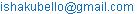(I. Bello)(M. B. Umar)(H. O. Idakwo)

*Corresponding author

Ishaku Bello, Musa Bukar Umar, Harrison Oyibo Idakwo. Determination of Bus Voltages, Real and Reactive Power Losses in the Northern Nigeria 330Kv Network Using Power System Analysis Tool (PSAT). Science Journal of Energy Engineering. Vol.4, No. 5, 2016, pp. 35-43. doi: 10.11648/j.sjee.20160405.11

Received: September 16, 2016; Accepted: October 27, 2016; Published: November 17, 2016

Abstract: The power system analysis and design is generally done by using Load flow studies. The essence of power flow analysis is to find the magnitude and phase angle of voltage at each bus and the real and reactive power flows in each transmission lines. Therefore, load flow analysis is an important tool involving numerical analysis applied to a power system. This analysis is being executed at the stage of planning, operation, control and economic scheduling. Load flow analysis is performed on a symmetrical steady state operating condition of a power system under normal operating condition. This information is essential for the continuous monitoring of the current state of the system and for analyzing the effectiveness of alternative plans for future system expansion to meet increased load demand. For power flow analysis, this research considered the 330KV of northern Nigeria transmission network with a view of estimating the real and reactive power flows, power losses in the entire network and phase angle using Power System Analysis Toolbox (PSAT).

Keywords: Load Flow Analysis, Transmission Network, PSAT, Bus Voltages, Power Losses

1. Introduction

Due to the above, new strategies, new methods of analysis and design are being sought to improve performance of power system.

According to : in their work on power flow analysis of the Nigerian transmission system, concluded that incorporating FACTS devices are adequately enough to improve the power demand of the country.

Also in : they identify poor power magnitude at Gombe, Kano, Jos, Kaduna and Katampe transmission station. Voltage violations were also improved by adding shunt capacitor compensators to the affected buses. The northern Nigeria is expanding with increase in agriculture output as a result more industries are coming up, adding shunt capacitor compensators can be a lasting solution to this problem.

In : they study the steady state voltage stability enhancement using SSSC, upon running load flow, they discover that some buses recorded voltage drop, but upon incorporating SSSC device there was significant improvement.

According to : he run a load flow analysis of 230/110kv substation using ETAP, he end up by recommending only capacitor bank for the correction of under voltage.

According to : they look at the behavior of the national grid before and after reform (unbundling of the power sector and the recent concluded/abandon project), the system has great improvement with the addition more generating power plant and more 330kv transmission network. The work depends heavily on assume data because up to now most of the generating plant and the transmission lines are under construction.

Much research is still ongoing in the determination of bus voltages, real and reactive power losses. It is the aim of this paper to investigate bus voltages, real and reactive power losses and flows on the northern Nigeria 330kv network using PSAT.

In  a simple and efficient computer algorithm has been presented to solve unbalanced radial distribution networks. The proposed method has good convergence property for any practical distribution networks with practical R/X ratio. Computationally, this method is extremely efficient.

2. Review of Current Nigeria Integrated Power System

2.1. Nigerian Electric Power Transmission System

The Nigerian electric power transmission network, operated by the Transmission Company of Nigeria (TCN), operates at a high pressure of 330kV while its lower transmission pressure is 132kV. The grid is an integrated network consisting of seventeen generating stations and fifty two 330kV bus; two of the generating stations (Trans Amadi G. S. and Omoku G. S.) are run on island operation. Only three generating stations (Jebba, Kainji and Shiroro Hydro power stations) and eleven transmitting stations were cited in the northern part of the country as at data collection time. Three of these transmitting stations (Damaturu T.S., Maiduguri T.S., and Yola T.S.) had no record of operation as at data collection time .

2.2. The Northern Nigeria Grid System

The northern Nigerian power system of 330kV transmission lines is the study area in the context of this work. It consists of 3 generator and 13 bus networks. All the three (3) generators are hydro units.

2.3. Power Balance Equations

As shown in equation (1) and (2), the total generation of real and reactive power must be equals to the total load demand plus losses, [4,10].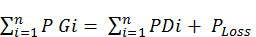(1)(2)

2.4. Power Flow Equations

Consider a typical bus of a power system network as shown in Figure 1.0. Transmission lines are represented by their equivalent π models where impedances have been converted to per – unit admittances on a common MVA base.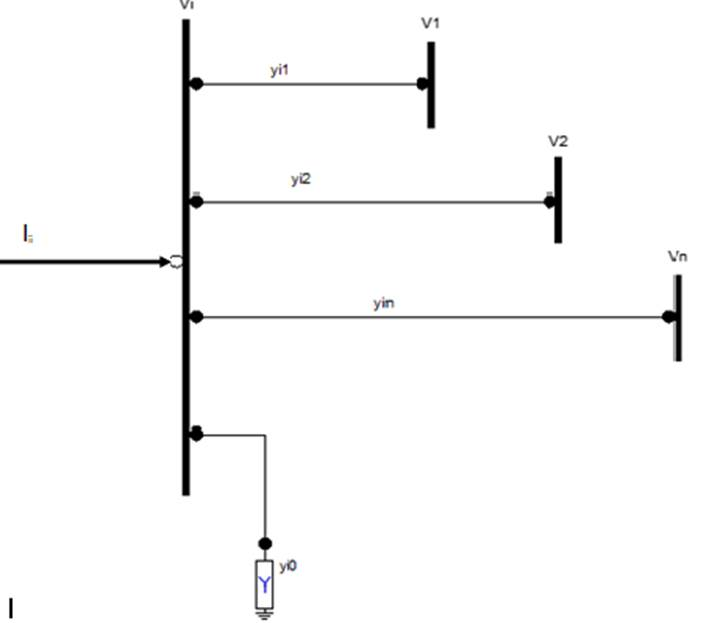Figure 1. Typical bus of the power system.

Application of Kirchhoff’s current Law (KCL) to this bus results in

Ii = yi0 Vi + yi1 (Vi - V1) + yi2 (Vi - V2) +......... yin (Vi - Vn)

= (yi0 + yi1 + yi2 + ....+ yin)Vi - yi1V1 - yi2V2 - ....... - yinVn   (3)

Ii = Vi-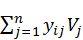, J I        (4)

The apparent power at bus i is

Si = Pi + jQi = ViI*i                  (5)

Or

Ii =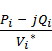(6)

Using equation (6) in equation (5) gives

Ii == Vi-, j I    (7)

From equation (8), the mathematical formulation of the power flow problem results in a system of algebraic non-linear equations which must be solved by iterative techniques.

2.4.1. Gauss - Seidel Power Flow Solution

In the power flow study, it is necessary to solve the set of non – linear equations represented by equation (8). In the Gauss – Seidel method equation (8) is solved for Vi and the iterative sequence becomes,

V(k+1) =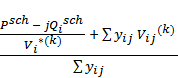j ≠ I        (8)

In writing the KCL, current entering bus i was assumed positive. Thus, for buses where real and reactive powers are injected into the bus, such as generator buses, Psch and Qsch have positive values. For load buses where real and reactive powers are flowing away from the bus, Psch and Qsch have negative values. If equation (5) is solved for Pi and Qi, we have

Pi(k+1) = Real[ Vi*(k){Vi(k)-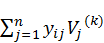}] J ≠ I  (9)

Qi(k+1) = Imaginary[ Vi*(k){Vi(k)-}] j ≠ I (10)

The bus admittance matrix Ybus is an important network description of the interconnected power system and the power flow equation is usually expressed in terms of the elements of the bus admittance matrix,Ybus. Since the off diagonal elements of the bus admittance matrix Ybus are Yij = - yij and the diagonal elements are Yii = ∑yij then equation (6) above becomes

V(k+1) =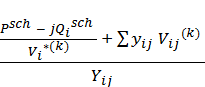(11)

And equation (10) and (11) becomes

Pi(k+1) = Real (Vi*(k){Vi(k)Yii +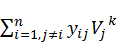}) j≠ i  (12)

Qi(k+1) = Imaginary(Vi*(k){Vi(k)Yii +}) j≠I (13)

Yii includes the admittance to ground of line charging susceptance and any other fixed admittance to ground.

2.4.2. Newton - Raphson Method

The load flow problem can also be solved by using Newton Raphson method. For the typical bus of the power system shown in Fig. 1.0 the current entering bus written in terms of the bus admittance matrix as.

Ii =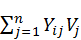(14)

Expressing equation (15) in polar form, we have

Ii =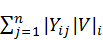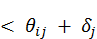(15)

The complex power at bus i is

Pi - jQi = Vi*Ii              (16)

Substituting equation (16) into equation (17)

Pi - jQi = |Vi| < -(17)

Separating the real and imaginary parts

Pi =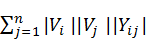cos (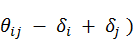(18)

Qi = -sin ((19)

Equation (19) and (20) constitute a set of non – linear algebraic equations in terms of the independent variables, voltage magnitude in per – unit and phase angle in radians. We have two equations for each load bus given by (19) and (20), and one equation for each voltage controlled bus, given by (19). Expanding (19) and (20) in Taylor’s series about the initial estimate and neglecting all higher order terms results in a set of linear equations. These equations after linearization can be written in matrix form as.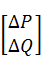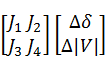(20)

Where element J1, J2, J3, J4 are elements of Jacobian matrix. In obtaining the power flow solution by Newton - Raphson method, we have to consider equation (21).

The diagonal and off diagonal element of J1 are: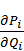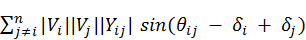(21)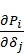= -|Vi||Vj||Yij|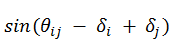j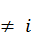(22)

The diagonal and off diagonal element of J2 are: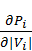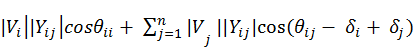(23)

The diagonal and off diagonal elements of J3 are: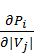=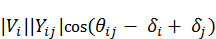j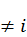(24)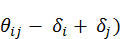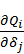=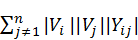cos ((25)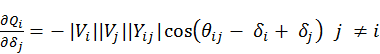(26)

The diagonal and off diagonal element of J4 are: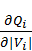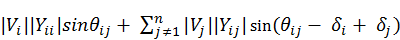(27)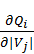= -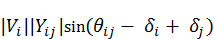j(28)

The terms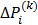and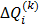are the differences between the scheduled and calculated values, known as the power residuals given by: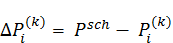(29)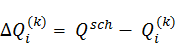(30)

The new estimates for bus voltages are: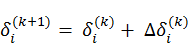(31)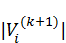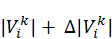(32)

2.4.3. Fast Decouple Newton- Raphson Power Flow Solution

This is an extension of Newton – Raphson method formulated in polar coordinates with certain approximation which results in fast algorithm for load flow solution. Because power system transmission lines have a very high X/R ratio thus it is reasonably assumed that real power changes (ΔP) are less sensitive to changes in voltage magnitude and are mainly sensitive to changes in phase angle (Δ). Similarly, the reactive power is less sensitive to changes in phase angle Δbut mainly sensitive to changes in voltage magnitude. With these assumptions, recall equation (21)(33)

Or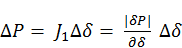(34)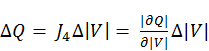(35)

Equations (31) and (32) show that the matrix equation is separated into two decoupled equations requiring considerably less time to solve compared to the time required for the solution of (19). Furthermore, considerable simplification can be made to eliminate the need for re-computing J1and J4 during each iteration. This procedure however, results in Fast Decoupled power flow equations .

3. Methodology

The design in the context of this paper will be limited to the northern Nigeria 330Kv network using PSAT, in other to achieve the following aim;

i.  To determine bus voltages, real and reactive power losses and flows on the northern Nigeria 330kv network using PSAT

ii.   To design a model that can be used to determine the actual calculation of active and reactive power.

iii.   To carry out an in-depth studies on load flow analysis.

iv.   To evaluate the performance of the model on the collected data.

v.   To design a model that can be used to plan ahead the load demand by consumers.

3.1. Data Collection

The data used in this analysis and assessment were collected from Transmission company of Nigeria (TCN). These was modeled and simulated in PSAT 2.1.9 using N-R power flow algorithm. The network for this study consist of Three (3) generating stations, Thirteen (13) buses, Nine (9) loads and Twelve(12) transmission lines using N-R and modeled with Power System Analysis Toolbox (PSAT). Simulation of the designed model was carried out in other to determine the active and reactive power flows in all branches in a network, active and reactive power contributed by each generator, active and reactive power losses in each component in the network, bus voltages magnitudes and angles throughout the network.

3.2. Design and Simulation of Northern Nigeria 330KV Network using N-R Method

The Newton-Raphson method formulates and solves iteratively the following load flow equation (15-33).

Recall equation (21)(21)

Where ΔP and ΔQ are bus real power and reactive power mismatch vectors between specified value and calculated value, respectively; ΔV and Δrepresents bus voltage angle and magnitude vectors in an incremental form; and J1 through J4 are called Jacobian matrices. The Newton Raphson method possesses a unique quadratic convergence characteristic. It usually has a very fast convergence speed compared to other load flow calculation methods. It also has the advantage that the convergence criteria are specified to ensure convergence for bus real power and reactive power mismatches. This criterion gives the direct control of the accuracy method of Newton-Raphson. The convergence criteria for the Newton-Raphson method are typically set to 0.001MW and MVar. The Newton-Raphson method is highly dependent on the voltage initial values. Flow Chart for Newton-Raphson Algorithm used for the Northern Nigeria 330KV Network.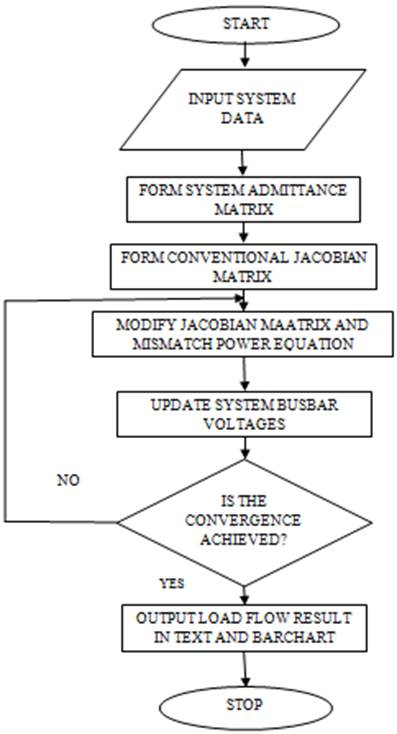Figure 2. Flow chart for Newton-Raphson Algorithm.

3.3. IEEE 14 Bus Test Network

Test network system is widely used in power system research and education. It is imperative to understand the importance of using the standard test network. This is very vital because; Practical power systems data are partially confidential, also the dynamic and static data of the system are not well documented, more so, Calculations of numerous scenarios are difficult due to large set of data and the lack of software capabilities for handling large set of data less generic results from practical power system.

The 14 bus system consists of five synchronous machines with IEEE type; 1 exciter, four of which are synchronous compensators used only for reactive power support. There are nine load buses in the system totaling to 259MW and 81.3 MVAR. The dynamic and static data of the system can be found. The system is widely used for voltage stability as well as low frequency oscillatory stability analysis. The 14 bus test case does not have line limits compared to other systems. It has also a low base voltage and an overabundance of voltage control capability.

4. Results and Discussion

The result obtained in this section shows the power flows in the transmission lines and losses from both generators and lines. The bus voltages were also obtained to know the weak ones among them. Comparism was made between the Northern Nigeria grid and the IEEE 14 bus test system.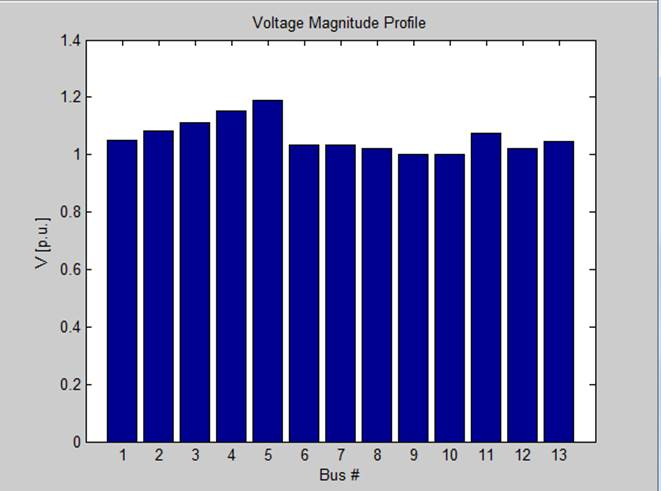Figure 3. Voltage magnitude profile of Northern Nigeria.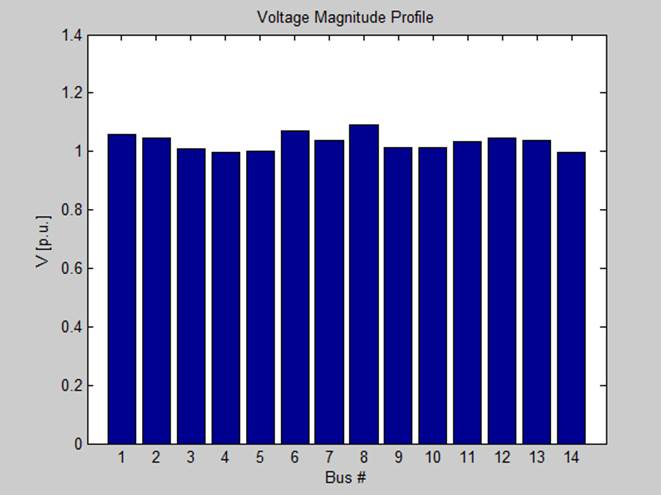Figure 4. Voltage magnitude profile of IEEE 14 bus test.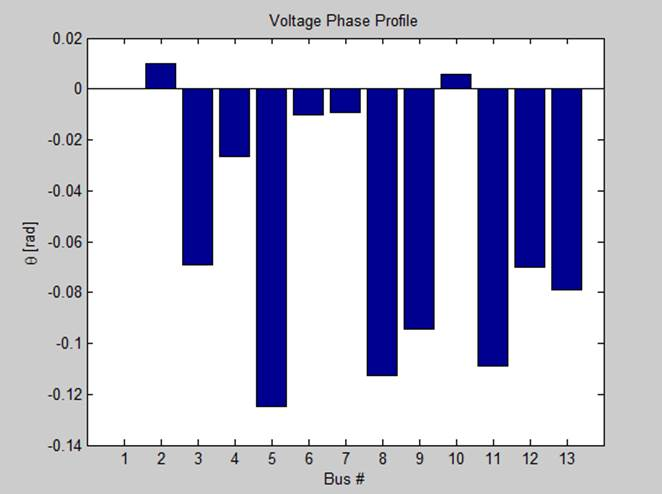Figure 5. Voltage phase Profile of Northern Nigeria.Figure 6. Voltage Phase Profile of IEEE 14 bus test.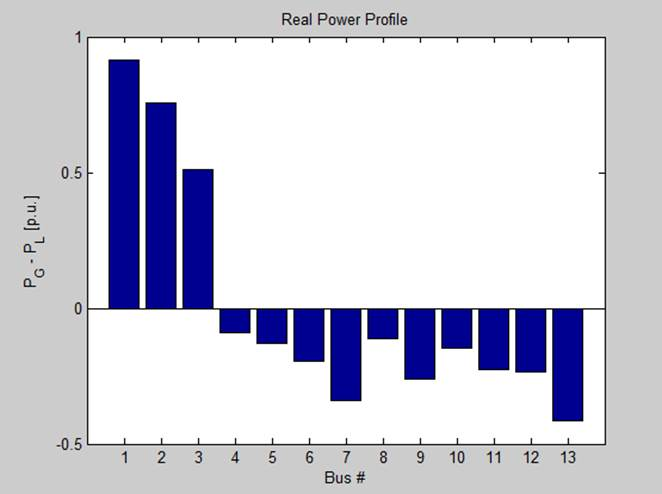Figure 7. Real Power Profile of Northern Nigeria.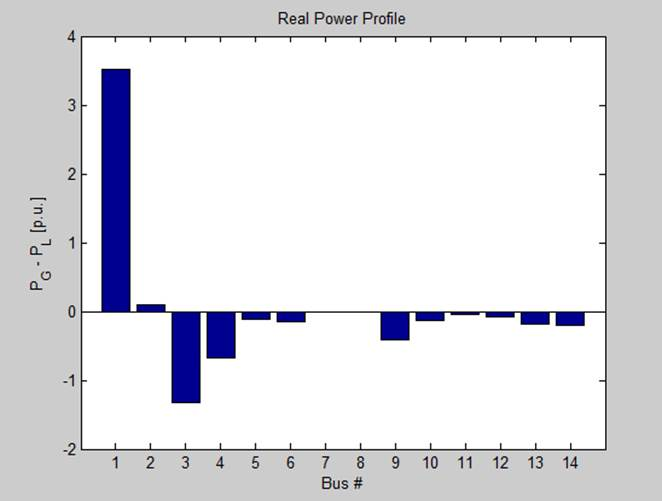Figure 8. Real power profile of IEEE 14 bus test.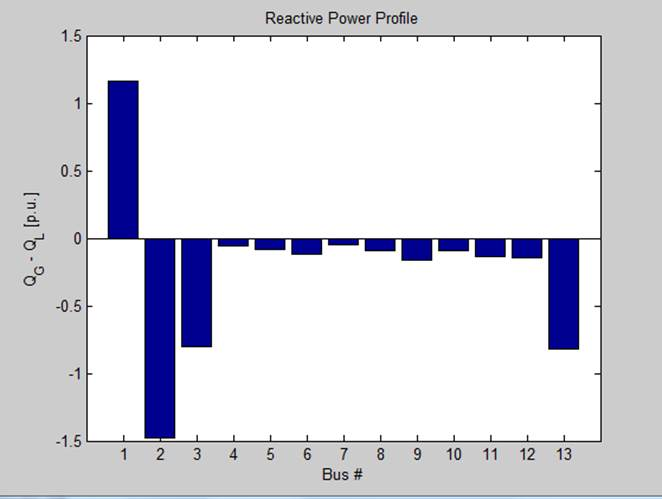Figure 9. Reactive power profile of Northern Nigeria.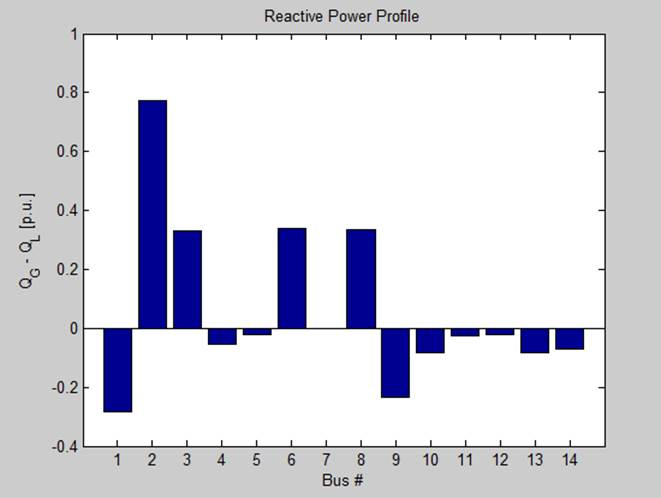Figure 10. Reactive power profile of IEEE 14 bus test.

Table 1. Solution Statistics.

 DESCRIPTION OF ITEM IEEE 14 BUS NORTHERN NIGERIA Number of Iterations 9 4 Maximum P Mismatch [p.u] 0 0 Maximum Q Mismatch [p.u] 0 0 Power rate [MVA] 100 100

Table 2. Power Flow Result of Northern Nigeria.

 Bus V [P.u] Phase [rad] PGen [P.u] QGen [P.u] PLoad [P.u] QLoad [P.u] Bus 01 1.05000 0.00000 0.91476 1.1616 0.00000 0.00000 Bus 02 1.00100 0.00994 0.75600 -1.4766 0.00000 0.00000 Bus 03 1.02100 -0.06914 0.51300 -0.8082 0.00000 0.00000 Bus 04 1.03400 -0.02651 0.00000 0.00000 0.08900 0.05500 Bus 05 1.11480 -0.12489 0.00000 0.00000 0.13000 0.08000 Bus 06 1.03280 -0.01000 0.00000 0.00000 0.19400 0.12000 Bus 07 1.03510 -0.00915 0.00000 0.00000 0.33900 0.04900 Bus 08 1.09950 -0.11266 0.00000 0.00000 0.11400 0.09000 Bus 09 1.05290 -0.09417 0.00000 0.00000 0.26000 1.16100 Bus 10 1.00470 0.00575 0.00000 0.00000 0.14600 0.09000 Bus 11 1.05710 -0.10890 0.00000 0.00000 0.22600 0.14000 Bus 12 1.02040 -0.07080 0.00000 0.00000 0.23600 0.14600 Bus 13 0.99807 -0.07898 0.00000 0.00000 0.41300 0.82300

Table 3. Power Flow Result IEEE 14-Bus Test System.

 Bus V [P.u] Phase [rad] PGen [P.u] QGen [P.u] PLoad [P.u] QLoad [P.u] Bus 01 1.06000 0.00000 3.35730 0.78985 0.00000 0.00000 Bus 02 1.00090 -0.11932 0.57784 0.30000 0.30380 0.17780 Bus 03 0.93206 -0.33419 0.00000 0.40000 1.31880 0.26600 Bus 04 0.93066 -0.25988 0.00000 0.00000 0.66920 0.05600 Bus 05 0.94205 -0.22069 0.00000 0.00000 0.10640 0.02240 Bus 06 0.96224 -0.39544 0.00000 0.24000 0.15680 0.10500 Bus 07 0.94209 -0.36556 0.00000 0.00000 0.00000 0.00000 Bus 08 0.98501 -0.35556 0.00000 0.24000 0.00000 0.00000 Bus 09 0.91460 -0.42345 0.00000 0.00000 0.41300 0.02324 Bus 10 0.91087 -0.42733 0.00000 0.00000 0.12600 0.08120 Bus 11 0.93046 -0.41503 0.00000 0.00000 0.04900 0.02520 Bus 12 0.93675 -0.42244 0.00000 0.00000 0.08540 0.02240 Bus 13 0.92694 -0.42495 0.00000 0.00000 0.18900 0.08120 Bus 14 0.89044 -0.45658 0.00000 0.00000 0.20860 0.07000

Table 4. Line Flows of Northern Nigeria.

 From Bus To Bus Line PFlow [P.u] QFlow [P.u] PLoss [P.u] QLoss [P.u] Bus 06 Bus 07 1 -0.57320 -1.15370 0.00031 -0.00739 Bus 06 Bus 10 2 -0.51126 1.20420 0.00577 -0.12186 Bus 10 Bus 04 3 0.08968 -0.24788 0.00068 -0.30288 Bus 06 Bus 12 4 0.89046 -0.17058 0.0072 -0.45108 Bus 12 Bus 13 5 0.41568 0.65089 0.000268 -0.17211 Bus 09 Bus 12 6 -.073601 1.17010 0.00744 -0.14694 Bus 09 Bus 11 7 0.22651 -0.35827 0.00051 -0.49827 Bus 08 Bus 09 8 -0.24494 0.54136 0.00456 -0.43144 Bus 05 Bus 08 9 -0.13000 -0.08000 0.000094 -0.71136 Bus 01 Bus 07 10 0.91476 1.16160 0.00225 -0.03363 Bus 02 Bus 10 11 0.75600 -1.47660 0.00329 -0.0074 Bus 03 Bus 12 12 0.51300 -0.80282 0.00113 -0.00221

Table 5. Line Flows of IEEE 14 Bus Network Systems.

 From Bus To Bus Line PFlow [P.u] QFlow [P.u] PLoss [P.u] QLoss [P.u] Bus 02 Bus 05 1 0.604090 0.15190 0.022370 0.036170 Bus 06 Bus 12 2 0.115200 0.04181 0.000199 0.004150 Bus 12 Bus 13 3 0.027800 0.01526 0.000125 0.000230 Bus 06 Bus 13 4 0.267080 0.12811 0.006270 0.012340 Bus 06 Bus 11 5 0.131930 0.09165 0.002650 0.005540 Bus 11 Bus 10 6 0.080280 0.06090 0.000960 0.002250 Bus 09 Bus 10 7 0.046790 0.02282 0.000100 0.000270 Bus 09 Bus 14 8 0.113800 0.02987 0.002100 0.004470 Bus 14 Bus 13 9 -0.096900 -0.04460 0.002450 0.004990 Bus 07 Bus 09 10 0.452560 0.24844 0.000000 0.033040 Bus 01 Bus 02 11 2.278400 0.40970 0.092870 0.227430 Bus 03 Bus 02 12 -0.999710 0.00238 0.054080 0.186890 Bus 03 Bus 04 13 -0.319090 0.13162 0.009510 -0.005700 Bus 01 Bus 05 14 1.078800 0.38014 0.063960 0.214570 Bus 05 Bus 04 15 0.819200 0.00542 0.010100 0.020630 Bus 02 Bus 04 16 0.801700 0.16807 0.039300 0.084320 Bus 04 Bus 09 17 0.121150 0.08266 0.000120 0.012970 Bus 05 Bus 06 18 0.671000 0.25348 0.000000 0.126910 Bus 04 Bus 07 19 0.452560 0.06724 0.000000 0.048340 Bus 08 Bus 07 20 0.000000 0.24000 0.000000 0.010460

4.1. Discussion of Result

It can be seen that from figure 3 Bus 05, Bus 09, and Bus 11 have exceed the voltage limit of 1.05 p.u and the reactive power generated by generator 2 at Bus 02 and generator 3 at Bus 03 are high. Base on the global summary, Real power generated was 218.38MW (2.1838 p.u) and total reactive power of -111.78Mvar (-1.1178 p.u) and total Load demand was Real power 214.7MW (2.147 p.u) and Reactive power 175.4 Mvar (1.754 p.u) and total losses due to transmission line was Real power 3.678 MW (0.003676 p.u), Reactive power -287.18 Mvar (-2.8718 p.u). The reactive power loss is higher than the reactive power generated. From table 4 it can be seen that between Bus 05 to Bus 08 i.e. Line 9 the reactive power loss is high and between Bus 09 to Bus 12 i.e. Line 6 has the highest real power loss of 0.744 MW.

4.2. Conclusion

From the result discussed, one can say that the power generated in Northern Nigeria is not adequate to meet the increasing demand. However, the available power generated also suffers losses due to ageing transmission lines and other power equipment.

Acknowledgements

Special Thanks goes to Power Holding Company for supplying us with the data needed for this research work.

References

1. Sambo A. S, Garba B., I. H Zarma and M. M. Gaji, " Electricity Generation and Present challenges in the Nigerian Power Sector". Unpublished paper, Energy Commission of Nigeria, Abuja-Nigeria, 2010.
2. Onohaebi S. O and Omorogiuwa Eseosa "Smart Grid and Energy Management in Nigeria Integrated Power System". International Journal of Engineering Innovation & Research Vol.3, issue 6, ISSN: 2277-5668 October 2014.
3. E. Acha., V. G. Agelidis, O. Anaya - Lara, T. J. E. Miller., "Power Electronics Control in Electrical Systems" Newness Power Engineering Series 2002.
4. Adepoju G. A, Komolafe O. A, Aborishade D. O. "Power Flow Analysis of the Nigerian Transmission System Incorporating Facts Controllers". International Journal of Applied Science and Technology. vol. 1 No. 5; September 2011.
5. Adebayo I. G, Aborishade D. O, and Oyesina K. A." Steady State Voltage Stability Enhancement Using Static Synchronous Series Compensator (SSSC); A Case Study of Nigerian 330KV Grid System" RJEAS 2013.
6. Rajesh krishnasamy, Karuppasamypandiyan M. and A. Bhuvanesh"Power Flow Analysis of 230/110KV Substation using ETAP" Conference paper February 2014. ResearchGate.
7. O. J Onojo, G. C Ononiwi and S. O Okozi. "Analysis of Power Flow of Nigerian 330KV Grid System (Pre and Post) Using Matlab" EJNAS. Vol. 1, issue 2, 2013.
8. Aminu A. Maraf and Kangiwa U. Garba" Determination of Bus Voltages, Power Losses and Load Flow in the Northern Nigerian 330KV Transmission Sub-Grid" IJART Vol. 2, issue 3, March 2013.
9. Nirbhaykumar N., "Methods for Load Flow Analysis of Weakly Meshed Distribution System Makwana"International Journal of Scientific and Research Publications, Volume 2, Issue 3, March 2012.

 Contents 1. 2. 2.1. 2.2. 2.3. 2.4. 3. 3.1. 3.2. 3.3. 4. 4.1. 4.2.
Article ToolsAbstractPDF(457K)